JMSLTM Numerical Library 7.2.0
com.imsl.datamining.neural

## Class TimeSeriesFilter

• All Implemented Interfaces:
Serializable

```public class TimeSeriesFilter
extends Object
implements Serializable```
Converts time series data to a lagged format used as input to a neural network.

Class `TimeSeriesFilter` can be used to operate on a data matrix and lags every column to form a new data matrix. Using the method `computeLags`, each column of the input matrix, `x`, is transformed into (`nLags`+1) columns by creating a column for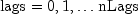.

The output data array, z, can be symbolically represented as: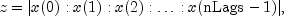where `x(i)` is a lagged column of the incoming data matrix, `x`.

Consider, an example in which `x` has five rows and two columns with all variables continuous input attributes. Using nObs and nVar to represent the number of rows and columns in `x`, let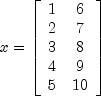If `nLags=1`, then the number of columns in z[][] is nVar*(nLags+1)=2*2=4, and the number of rows is (nObs-nLags)=5-1=4: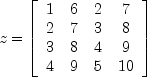If `nLags=2`, then the number of rows in z will be (nObs-nLags)=(5-2)=3 and the number of columns will be nVar*(nLags+1)=2*3=6: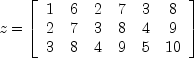Example, Serialized Form
• ### Constructor Summary

Constructors
Constructor and Description
`TimeSeriesFilter()`
Constructor for `TimeSeriesClassFilter`.
• ### Method Summary

Methods
Modifier and Type Method and Description
`double[][]` ```computeLags(int nLags, double[][] x)```
Lags time series data to a format used for input to a neural network.
• ### Methods inherited from class java.lang.Object

`clone, equals, finalize, getClass, hashCode, notify, notifyAll, toString, wait, wait, wait`
• ### Constructor Detail

• #### TimeSeriesFilter

`public TimeSeriesFilter()`
Constructor for `TimeSeriesClassFilter`.
• ### Method Detail

• #### computeLags

```public double[][] computeLags(int nLags,
double[][] x)```
Lags time series data to a format used for input to a neural network.
Parameters:
`nLags` - An `int` containing the requested number of lags. `nLags` must be greater than 0.
`x` - A `double` matrix, nObs by nVar, containing the time series data to be lagged. It is assumed that `x` is sorted in descending chronological order.
Returns:
A `double` matrix with (nObs-`nLags`) rows and (nVar(`nLags+1`)) columns. The columns 0 through (nVar-1) contain the columns of `x`. The next nVar columns contain the first lag of the columns in `x`, etc.
JMSLTM Numerical Library 7.2.0